2019-05-02 09:44:40 slozer 阅读数 128
• ###### 深度学习基础与TensorFlow实践教程

本课程主要介绍了深度学习的基础原理和TensorFlow系统基本使用方法。TensorFlow是目前机器学习、深度学习领域优秀的计算系统之一，本课程将结合实例介绍使用TensorFlow开发机器学习应用的详细方法和步骤，着重讲解了用于图像识别的卷积神经网络和用于自然语言处理的循环神经网络的理论知识及其TensorFlow实现方法，并结合实际场景和例子描述了深度学习技术的应用范围与效果。 所有案例均来自讲师团队工作中的亲身实践，所选案例均是深度学习的经典应用，非常具有代表性。

21447 人正在学习 去看看 AI100讲师

CNTK是微软出品的一个开源的深度学习工具包，Tensorflow就不加以阐述;这两个类库虽然强大，但实际运用中直接使用是非常困难的。本文会介绍一个构建在CNTK和Tensorlow上的，用来快速创建深度学习模型的python类库，就是keras

### 安装问题

1.按照书上的指示 ，在终端执行如下操作

 pip install …


2.但是这样就报错

…is not a supported  wheel on this platform


import pip._internal
print(pip.internal.pep425tags.get_supported())


3.选择在原有anaconda中安装py36版本（anaconda支持多个py版本安装）

conda create -n py36 python=3.6 anaconda


4.将清华镜像站去除，换为中科大镜像站后发现不能移出

5.在终端输出python，显示py37版本，后进入tensorflow中，发现py36版本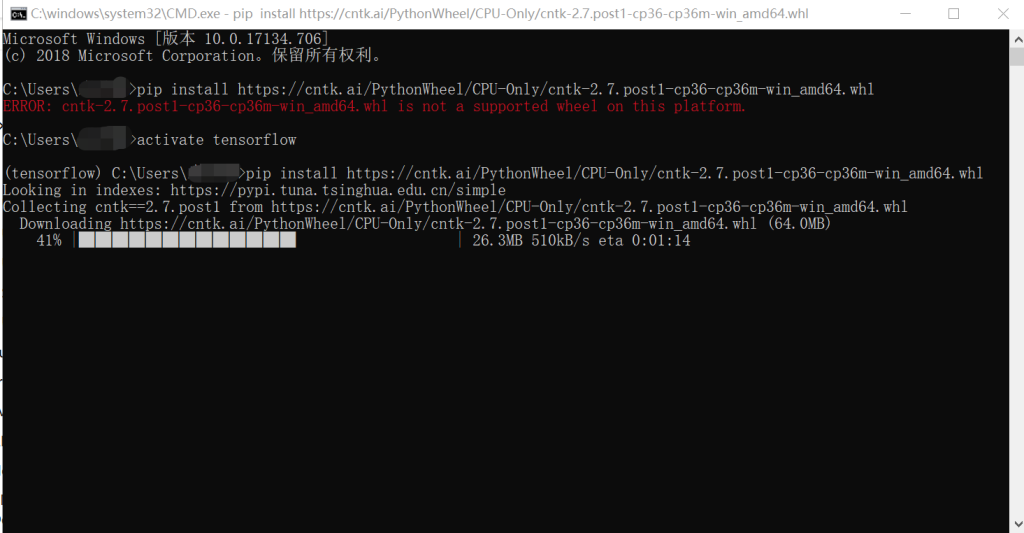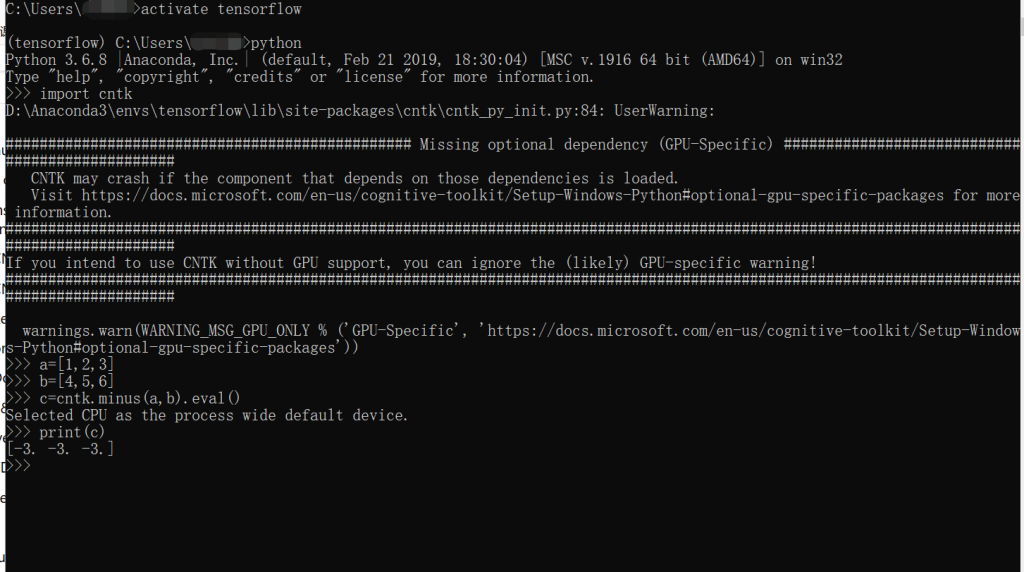6.ketas安装无问题，按照书上指示即可。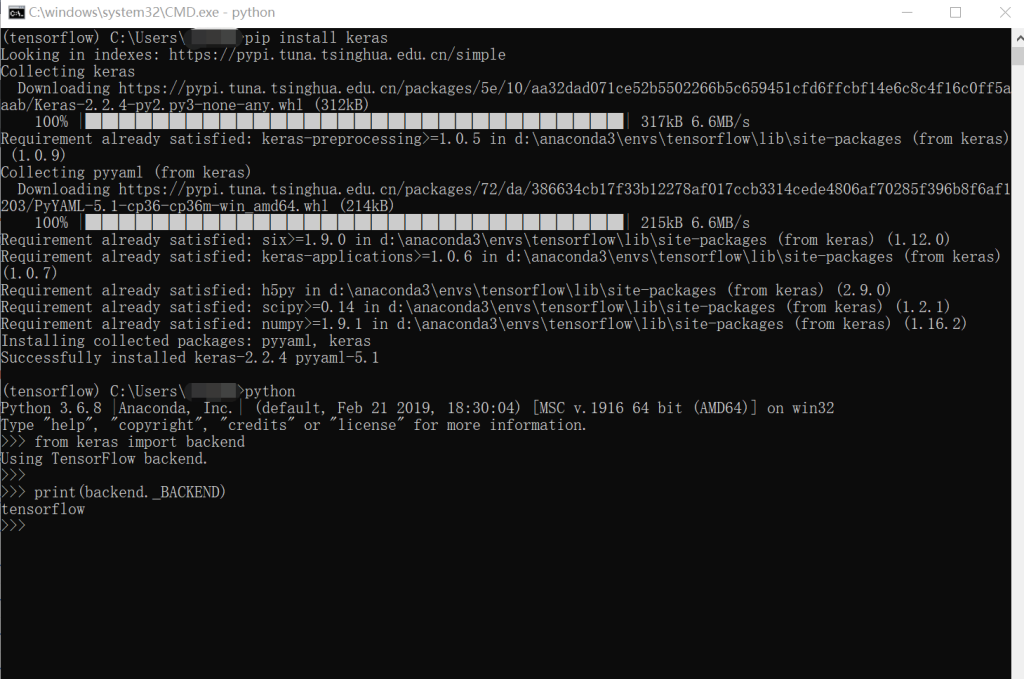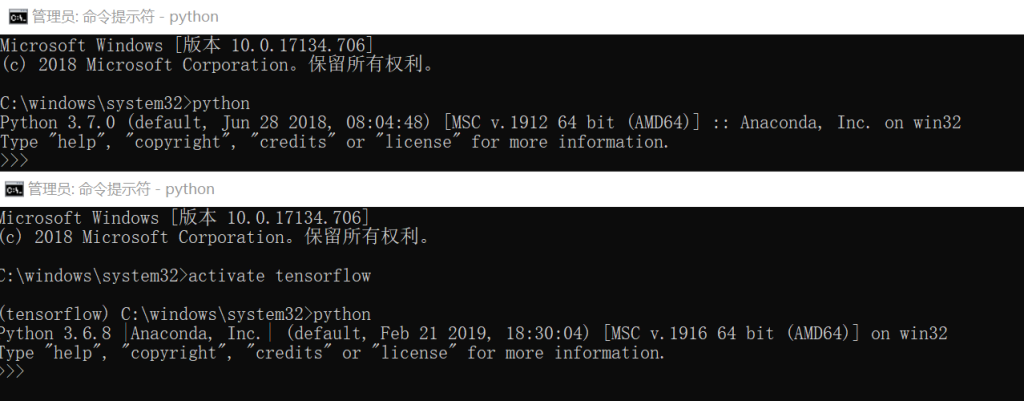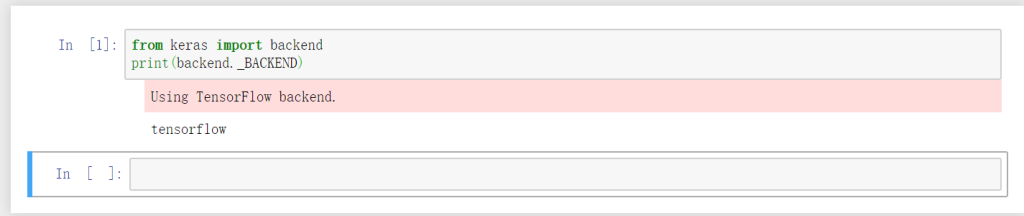### 例子

1.印第安人糖料病诊断
2.手写数字识别
3.爱丽丝梦游仙境

2017-09-14 16:55:00 weixin_34186128 阅读数 34
• ###### 深度学习基础与TensorFlow实践教程

本课程主要介绍了深度学习的基础原理和TensorFlow系统基本使用方法。TensorFlow是目前机器学习、深度学习领域优秀的计算系统之一，本课程将结合实例介绍使用TensorFlow开发机器学习应用的详细方法和步骤，着重讲解了用于图像识别的卷积神经网络和用于自然语言处理的循环神经网络的理论知识及其TensorFlow实现方法，并结合实际场景和例子描述了深度学习技术的应用范围与效果。 所有案例均来自讲师团队工作中的亲身实践，所选案例均是深度学习的经典应用，非常具有代表性。

21447 人正在学习 去看看 AI100讲师相关厂商内容那么如何实现Cloud Machine Learning平台呢？架构上进行了分层抽象，实现上也只需要三步。目前先知平台主要解决以下几个目标场景：

1. 简化数据引入，平台不要求数据必须使用分布式存储，也可以直接从RDBMS这类的SQL数据库中导入训练样本数据。

2. 简化数据拆分，用户不需要写Spark代码，只需要提供数据拆分后的存储路径，并且支持按比例拆分或者按规则拆分两种模式。

3. 简化特征抽取，平台支持连续特征、离散特征的抽取和组合，对于连续特征支持自动化的多分桶算法，我们也会归纳常用的特征抽取方法并且封装成脚本或者配置，用户只需了解对应的配置而不需要自己编码实现具体的逻辑。平台还可以根据已有的特征配置自动进行特征组合拓展，提升模型效果。

4. 简化模型训练，平台可以支持开源的机器学习算法实现和第四范式自主研发的超高维度LR算法，这个LR算法实现了Parameter server可以解决几十亿、上百亿特征维度的高速分布式训练问题。对于学习率、正则化参数等可以做到自动调优。另外，平台还提供了线性分形分类器等扩展算法，可以无须人为干预的更加有效利用数值类特征。

5. 简化模型评估，得到模型预估结果后，我们可以计算模型的ROC、Logloss、K-S等指标，不同模型计算指标的方式是类似的，用户就不需要重复编写实现代码，直接通过拖拽算子调用即可，以上都是先知平台所解决的问题。

6. 简化模型上线，对于常见的高维LR/GBDT模型，可以一键发布为线上服务实例，不仅简化了模型的部署和运维，而且上线实例还包括大部分的特征工程逻辑，无须手动再次开发特征处理逻辑的线上版本。2016-03-01 14:38:58 qq_33771258 阅读数 2729
• ###### 深度学习基础与TensorFlow实践教程

本课程主要介绍了深度学习的基础原理和TensorFlow系统基本使用方法。TensorFlow是目前机器学习、深度学习领域优秀的计算系统之一，本课程将结合实例介绍使用TensorFlow开发机器学习应用的详细方法和步骤，着重讲解了用于图像识别的卷积神经网络和用于自然语言处理的循环神经网络的理论知识及其TensorFlow实现方法，并结合实际场景和例子描述了深度学习技术的应用范围与效果。 所有案例均来自讲师团队工作中的亲身实践，所选案例均是深度学习的经典应用，非常具有代表性。

21447 人正在学习 去看看 AI100讲师

# Cntk学习日志（一）

·比如根据识别妹子的 语音，给妹子打分（萌妹子值99%,or 腐女70%）。

·比如水果识别，药品 识别，扫一扫就知道这是什么水果，含有那些维生素，或药品说明书。

·比如根据用户上传的 图片，识别出图片里的各种东西，根据图片里的东西编一个故事。

topoplot = [

action = "plot"

#这行命令是你所生成的神经网络的路径    modelPath="D:\CNTK\cntk\Examples\Other\Simple2d\Output\Models\Simple.dnn"

#生成dot文件

outputdotFile = "Output\lstm.model.dot"

#输出dot文件的位置

outputFile="Output\lstm.model.jpg"

#这行命令为你所下载的dot.exe文件路径加要生成图像的dot文件和图像的名称

renderCmd="D:\CNTK\cntk\cntk\dot\bin\dot.exe -Tjpg <IN> -Output\lstm.model.dot<OUT>-Output\lstm.model.jpg"

]

*文章未完，图为生成的神经网络图，由于笔者是小白，如文章有误，多谢指正。

2018-01-07 19:47:55 qq_34739497 阅读数 133
• ###### 深度学习基础与TensorFlow实践教程

本课程主要介绍了深度学习的基础原理和TensorFlow系统基本使用方法。TensorFlow是目前机器学习、深度学习领域优秀的计算系统之一，本课程将结合实例介绍使用TensorFlow开发机器学习应用的详细方法和步骤，着重讲解了用于图像识别的卷积神经网络和用于自然语言处理的循环神经网络的理论知识及其TensorFlow实现方法，并结合实际场景和例子描述了深度学习技术的应用范围与效果。 所有案例均来自讲师团队工作中的亲身实践，所选案例均是深度学习的经典应用，非常具有代表性。

21447 人正在学习 去看看 AI100讲师

## 前言

MNIST作为入门案例，我们继续以它作为实践例子来实践深度学习的模型。

## 卷积计算## LeNet-5 模型## 代码实现

mnist_infernece.py代码

import tensorflow as tf
INPUT_NODE=784#也就是图片28*28*1，由于只有一个通道
OUTPUT_NODE=10#输出分类
IMAGE_SIZE=28#输入图片像素大小
NUM_CHANNELS=1#通道数
NUM_LABELS=10#10个分类标签

CONV1_DEEP=32#第一个卷积层深度
CONV1_SIZE=5#卷积层的长宽

CONV2_DEEP=64#第二个卷积层深度
CONV2_SIZE=5#卷积层长宽

FC_SIZE=512#全连接层节点数

def get_weight_variable(shape,regularizer):#是否正则化
weights=tf.get_variable("weights",shape,initializer=tf.truncated_normal_initializer(stddev=0.1))
if regularizer !=None:
return weights

def inference(input_tensor,train,regularizer):#定义LeNet-5模型
with tf.variable_scope('layer1-conv1'):#命名空间定义第一个卷积层
conv1_weights=tf.get_variable("weight",[CONV1_SIZE,CONV1_SIZE,NUM_CHANNELS,CONV1_DEEP],initializer=tf.truncated_normal_initializer(stddev=0.1))#卷积层的参数定义成四维矩阵，由卷积层长宽，图片的通道数，由于手写图片是灰白，所以通道数始终是1，以及第一个卷积层深度构成。
conv1_biases=tf.get_variable("bias",[CONV1_DEEP],initializer=tf.constant_initializer(0.0))#偏差b
with tf.variable_scope('layer2-pool1'):#第一个池化层

with tf.variable_scope('layer3-conv2'):#命名空间定义第二个卷积层
conv2_weights = tf.get_variable(
"weight", [CONV2_SIZE, CONV2_SIZE, CONV1_DEEP, CONV2_DEEP],
initializer=tf.truncated_normal_initializer(stddev=0.1))
conv2_biases=tf.get_variable("bias",[CONV2_DEEP],initializer=tf.constant_initializer(0.0))

with tf.name_scope('layer4-pool2'):

pool_shape=pool2.get_shape().as_list()
nodes=pool_shape*pool_shape*pool_shape
reshaped=tf.reshape(pool2,[pool_shape,nodes])
with tf.variable_scope('layer5-fc1'):
fc1_weights=tf.get_variable("weight",[nodes,FC_SIZE],initializer=tf.truncated_normal_initializer(stddev=0.1))
if regularizer!=None:
fc1_biases=tf.get_variable("bias",[FC_SIZE],initializer=tf.constant_initializer(0.1))
fc1=tf.nn.relu(tf.matmul(reshaped,fc1_weights)+fc1_biases)
if train:
fc1=tf.nn.dropout(fc1,0.5)#dropout可以随机将节点置为0，可以避免过拟合

with tf.variable_scope('layer6-fc2'):
fc2_weights=tf.get_variable("weight",[FC_SIZE,NUM_LABELS],initializer=tf.truncated_normal_initializer(stddev=0.1))
if regularizer!=None:
fc2_biases=tf.get_variable("bias",[NUM_LABELS],initializer=tf.constant_initializer(0.1))
logit=tf.matmul(fc1,fc2_weights)+fc2_biases
return logit

mnist_train.py代码实现

import os
import tensorflow as tf
from tensorflow.examples.tutorials.mnist import input_data
import mnist_inference
import numpy as np
import pandas as pd
BATCH_SIZE=100#batch大小
LEARNING_RATE_BASE=0.01#学习率初始值
LEARNING_RATE_DECAY=0.99
REGULARAZTION_RATE=0.0001
TRAINING_STEPS=10000#迭代次数
MOVING_AVERAGE_DECAY=0.99
MODEL_SAVE_PATH="C:\\Users\\user\\Desktop"#永久化存储模型路径
MODEL_NAME="model.ckpt"
def train(mnist):
x=tf.placeholder(tf.float32,[BATCH_SIZE,mnist_inference.IMAGE_SIZE,mnist_inference.IMAGE_SIZE,mnist_inference.NUM_CHANNELS],name='x-input')#定义输入变量结构
y_=tf.placeholder(tf.float32,[None,mnist_inference.OUTPUT_NODE],name='y-input')

regularizer=tf.contrib.layers.l2_regularizer(REGULARAZTION_RATE)#采用L2正则化防止过拟合
y=mnist_inference.inference(x,False,regularizer)
global_step=tf.Variable(0,trainable=False)
variable_averages = tf.train.ExponentialMovingAverage(MOVING_AVERAGE_DECAY, global_step)#定义滑动平滑是预测更健壮
variables_averages_op = variable_averages.apply(tf.trainable_variables())
cross_entropy = tf.nn.sparse_softmax_cross_entropy_with_logits(logits=y,labels=tf.argmax(y_, 1))#定义交叉熵损失函数
cross_entropy_mean = tf.reduce_mean(cross_entropy)
learning_rate = tf.train.exponential_decay(
LEARNING_RATE_BASE,
global_step,
mnist.train.num_examples / BATCH_SIZE,
LEARNING_RATE_DECAY,
staircase=True)
with tf.control_dependencies([train_step, variables_averages_op]):
train_op = tf.no_op(name='train')

saver = tf.train.Saver()
with tf.Session() as sess:
tf.global_variables_initializer().run()

for i in range(TRAINING_STEPS):
xs,ys=mnist.train.next_batch(BATCH_SIZE)
reshaped_xs=np.reshape(xs,(BATCH_SIZE,mnist_inference.IMAGE_SIZE,mnist_inference.IMAGE_SIZE,mnist_inference.NUM_CHANNELS))
_, loss_value, step = sess.run([train_op, loss, global_step], feed_dict={x: reshaped_xs, y_: ys})

if i % 1000 == 0:
print("After %d training step(s), loss on training batch is %g." % (step, loss_value))
saver.save(sess, os.path.join(MODEL_SAVE_PATH, MODEL_NAME), global_step=global_step)
def main(argv=None):
train(data)

if __name__ == '__main__':
tf.app.run()mnist.eval.py代码实现

import time
import tensorflow as tf
from tensorflow.examples.tutorials.mnist import input_data
import mnist_inference
import mnist_train
import numpy as np
EVAL_INTERVAL_SECS=10
def evaluate(mnist):
with tf.Graph().as_default() as g:
x=tf.placeholder(tf.float32,[10000,mnist_inference.IMAGE_SIZE,mnist_inference.IMAGE_SIZE,mnist_inference.NUM_CHANNELS],name='x-input')
y_=tf.placeholder(tf.float32,[None,mnist_inference.OUTPUT_NODE],name='y-input')
xs,ys=mnist.train.next_batch(BATCH_SIZE)
reshaped_xs =  np.reshape(xs.values,(10000,mnist_inference.IMAGE_SIZE,mnist_inference.IMAGE_SIZE,mnist_inference.NUM_CHANNELS))
validate_feed={x:reshaped_xs,y_:ys}
y=mnist_inference.inference(x,False,None)
correct_prediction=tf.equal(tf.arg_max(y,1),tf.arg_max(y_,1))
accuracy=tf.reduce_mean(tf.cast(correct_prediction,tf.float32))
variable_averages=tf.train.ExponentialMovingAverage(mnist_train.MOVING_AVERAGE_DECAY)
variables_to_restore=variable_averages.variables_to_restore()
saver=tf.train.Saver(variables_to_restore)
while True:
with tf.Session() as sess:
ckpt = tf.train.get_checkpoint_state(mnist_train.MODEL_SAVE_PATH)
if ckpt and ckpt.model_checkpoint_path:
saver.restore(sess, ckpt.model_checkpoint_path)
global_step = ckpt.model_checkpoint_path.split('/')[-1].split('-')[-1]
accuracy_score = sess.run(accuracy, feed_dict=validate_feed)
print("After %s training step(s), validation accuracy = %g" % (global_step, accuracy_score))
else:
print('No checkpoint file found')
return
time.sleep(EVAL_INTERVAL_SECS)

def main(argv=None):
evaluate(data)
if __name__ == '__main__':
tf.app.run()2017-09-04 09:12:27 kwame211 阅读数 2617
• ###### 深度学习基础与TensorFlow实践教程

本课程主要介绍了深度学习的基础原理和TensorFlow系统基本使用方法。TensorFlow是目前机器学习、深度学习领域优秀的计算系统之一，本课程将结合实例介绍使用TensorFlow开发机器学习应用的详细方法和步骤，着重讲解了用于图像识别的卷积神经网络和用于自然语言处理的循环神经网络的理论知识及其TensorFlow实现方法，并结合实际场景和例子描述了深度学习技术的应用范围与效果。 所有案例均来自讲师团队工作中的亲身实践，所选案例均是深度学习的经典应用，非常具有代表性。

21447 人正在学习 去看看 AI100讲师

Computational Network Toolkit (CNTK) 是微软出品的开源深度学习工具包。本文介绍CNTK的基本内容，如何写CNTK的网络定义语言，以及跑通一个简单的例子。

根据微软开发者的描述，CNTK的性能比Caffe，Theano， TensoFlow等主流工具都要强。它支持CPU和GPU模式，所以没有GPU，或者神经网络比较小的实验，直接用CPU版的CNTK跑就行了。 其开源主页在 https://github.com/Microsoft/CNTK  它把神经网络描述成一个有向图的结构，叶子节点代表输入或者网络参数，其他节点计算步骤。 它支持卷积神经网络和递归神经网络。 由于CNTK刚推出不久，大众教程估计不多，而且bug估计也不少。我学习的时候，主要参考三个资料：

1 官方入门教程  https://github.com/Microsoft/CNTK/wiki/Tutorial  本文也主要以这里的教程为例

2 官方论坛 https://github.com/Microsoft/CNTK/issues

3 官方论文 http://research.microsoft.com/pubs/226641/CNTKBook-20160217..pdf  这个有150页，我是当作字典来用，遇到问题的时候就在里面搜

command=Train:Test
Train=[
        action="train"

NDLNetworkBuilder = [
...
]

SGD = [
...
]

...
]

]
 Test=[ ... ]CNTK用网络描述语言（network description language, NDL）描述一个神经网络。 简单的说，我们要描述输入的feature，输入的label，一些参数，参数和输入之间的计算关系，以及目标节点是什么。

NDLNetworkBuilder=[

run=ndlLR

ndlLR=[
# sample and label dimensions
SDim=$dimension$
LDim=1

features=Input(SDim, 1)
labels=Input(LDim, 1)

# parameters to learn
B0 = Parameter(4)
W0 = Parameter(4, SDim)

B = Parameter(LDim)
W = Parameter(LDim, 4)

# operations
t0 = Times(W0, features)
z0 = Plus(t0, B0)
s0 = Sigmoid(z0)

t = Times(W, s0)
z = Plus(t, B)
s = Sigmoid(z)

LR = Logistic(labels, s)
EP = SquareError(labels, s)

# root nodes
FeatureNodes=(features)
LabelNodes=(labels)
CriteriaNodes=(LR)
EvalNodes=(EP)
OutputNodes=(s,t,z,s0,W0)
]
]
features=Input(SDim, 1)     labels=Input(LDim, 1) 和 B0 = Parameter(4)  等可以想象成是在定义变量。 输入是列向量，CNTK里面的运算全是矩阵运算，所以就把输入当做只有一列的矩阵。  t0 = Times(W0, features) 是做矩阵乘法，t0把输入和权重相乘，z0 是在t0上面加了一个bias，
s0表示经过一个激活函数。 B0,W0,t0,z0,s0构成了隐层的操作，这里定义的隐层有4个节点。 t,z,s是输出层的操作，s就是输出节点的值。 框架定义好之后，还需要指定一些根节点，用来指定特殊的任务，例如 FeatureNodes=(features)  和LabelNodes=(labels)分别规定了输入和输出节点，CriteriaNodes 是训练的


设置训练算法
SGD = [
epochSize=0     #  每轮迭代使用的样例数，  =0 表示使用整个训练集
minibatchSize=25  # 训练25个样本就更新一次参数
learningRatesPerMB=0.1                # learning rates per MB
maxEpochs=50    #迭代50次
]


reader = [
#customDelimiter = " "
file = "Train.txt"
miniBatchMode = "partial"
verbosity = 1
randomize = "none"

features=[
dim = $dimension$
start = 0
]

labels=[
start = $dimension$              # skip $dimension$ elements before reading the label (i.e. the first two dimensions so we have "x1 x2 y" basically)
dim = 1                          # label has 1 dimension
labelType=regression
labelMappingFile = "SimpleMapping.txt"
]
]

Test=[
action="test"
file="Test.txt"
features=[
dim=2
start=0
]
labels=[
start=$dimension$
dim=1
labelDim=2
]
]
]

Output模块和Test的流程基本一样，只不过最后一个不是评估，而是把属于OutputNodes的值给输出到文件。 Output模块会指定一个输出目录 outputPath = "LR.txt" ， 输出的文件以“LR.txt”为前缀，再加上变量命作为文件名。例如"LR.txt.W0"。

# output the results
Output=[
action="write"
file="Test.txt"
features=[
dim=$dimension$
start=0
]
labels=[
start=2
dim=1
labelType=regression
]
]
outputPath = "LR.txt"       # dump the output as text
]

dumpNodeInfo  用来输出参数的值。这在调试中很有用，例如去看看网络的参数是如何变化的：

dumpNodeInfo=[
action=dumpnode
printValues=true
]

####################################################################
-6.67130613
####################################################################
EP=SquareError ( labels , s )
features=InputValue [ 2 x 1 {1,2} ]
labels=InputValue [ 1 x 1 {1,1} ]
LR=Logistic ( labels , s )
s=Sigmoid ( z )
t=Times ( W , features )
1.23924482 1.59913719
####################################################################
z=Plus ( t , B )# Copyright (c) Microsoft. All rights reserved.

# logistic regression cntk script -- Network Description Language

# which commands to run
command=Train:Output:dumpNodeInfo:Test

#required...
modelPath="Models/LR_reg.dnn"        # where to write the model to
deviceId=-1                                # CPU
dimension=2                 # input data dimensions

# training config
Train=[
action="train"
traceLevel = 1
NDLNetworkBuilder=[

run=ndlLR

ndlLR=[
# sample and label dimensions
SDim=$dimension$
LDim=1

features=Input(SDim, 1)
labels=Input(LDim, 1)

# parameters to learn
B0 = Parameter(4)
W0 = Parameter(4, SDim)

B = Parameter(LDim)
W = Parameter(LDim, 4)

# operations
t0 = Times(W0, features)
z0 = Plus(t0, B0)
s0 = Sigmoid(z0)

t = Times(W, s0)
z = Plus(t, B)
s = Sigmoid(z)

LR = Logistic(labels, s)
EP = SquareError(labels, s)

# root nodes
FeatureNodes=(features)
LabelNodes=(labels)
CriteriaNodes=(LR)
EvalNodes=(EP)
OutputNodes=(s,t,z,s0,W0)
]
]

SGD = [
epochSize=0                              # =0 means size of the training set
minibatchSize=25
learningRatesPerMB=0.1                # learning rates per MB
maxEpochs=50
]

# parameter values for the reader
#customDelimiter = " "
file = "Train.txt"
miniBatchMode = "partial"
verbosity = 1
randomize = "none"

features=[
dim = $dimension$
start = 0
]

labels=[
start = $dimension$              # skip $dimension$ elements before reading the label (i.e. the first two dimensions so we have "x1 x2 y" basically)
dim = 1                          # label has 1 dimension
labelType=regression
labelMappingFile = "SimpleMapping.txt"
]
]
]

# test
Test=[
action="test"
randomize = "none"
file="Test.txt"
features=[
dim=$dimension$
start=0
]
labels=[
start = $dimension$              # skip $dimension$ elements before reading the label (i.e. the first two dimensions so we have "x1 x2 y" basically)
dim = 1                          # label has 1 dimension
labelType=regression
labelMappingFile = "SimpleMapping.txt"
]
]
]

# output the results
Output=[
action="write"
file="Test.txt"
randomize = "none"
features=[
dim=$dimension$
start=0
]

labels=[
start = $dimension$              # skip $dimension$ elements before reading the label (i.e. the first two dimensions so we have "x1 x2 y" basically)
dim = 1                          # label has 1 dimension
labelType=regression
labelMappingFile = "SimpleMapping.txt"
]
]
outputPath = "LR.txt"        # dump the output as text
]

dumpNodeInfo=[
action=dumpnode
printValues=false
]